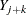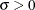# The MI Procedure

### MNAR Statement

• MNAR options;

The MNAR statement imputes missing values by using the pattern-mixture model approach, assuming the missing data are missing not at random (MNAR), which is described in the section Multiple Imputation with Pattern-Mixture Models. By comparing inferential results for these values to results for imputed values that are obtained under the missing at random (MAR) assumption, you can assess the sensitivity of the conclusions to the MAR assumption. The MAR assumption is questionable if it leads to results that are different from the results for the MNAR scenarios.

There are two main options in the MNAR statement, MODEL and ADJUST. You use the MODEL option to specify a subset of observations from which imputation models are to be derived for specified variables. You use the ADJUST option to specify an imputed variable and adjustment parameters (such as shift and scale) for adjusting the imputed variable values for a specified subset of observations.

The MNAR statement is applicable only if it is used along with a MONOTONE statement or an FCS statement. For a detailed explanation of the imputation process for the MNAR statement and how this process is implemented differently using the MONOTONE and FCS statements, see the section Multiple Imputation with Pattern-Mixture Models.

MODEL( imputed-variables / model-options )

specifies a set of imputed-variables in the VAR statement and the subset of observations from which the imputation models for these variables are to be derived. You can specify multiple MODEL options in the MNAR statement, but only one MODEL option for each imputed variable.

When an imputed variable that is listed in the VAR statement is not specified as an imputed-variable in the MODEL option, all available observations are used to construct the imputation for that variable.

The following model-options provide various ways to specify the subset of observations:

MODELOBS=CCMV <( K= k)>
MODELOBS=NCMV <( K= k)>
MODELOBS=( obs-variable=character-list)

identifies the subset of observations that are used to derive the imputation models.

When you use the MNAR statement along with an FCS statement, only the MODELOBS=( obs-variable=character-list) model-option is applicable. When you use the MNAR statement along with a MONOTONE statement, all three model-options are applicable.

MODELOBS=CCMV specifies the complete-case missing values method (Little 1993; Molenberghs and Kenward 2007, p. 35). This method derives the imputation model from the group of observations for which all the variables are observed.

MODELOBS=CCMV(K=k) uses the k groups of observations together with as many observed variables as possible to derive the imputation models. For a data set that has a monotone missing pattern and p variables, there are at most p groups of observations for which the same number of variables is observed. The default is K=1, which uses observations from the group for which all the variables in the VAR statement are observed (this corresponds to MODELOBS=CCMV).

MODELOBS=NCMV specifies the neighboring-case missing values method (Molenberghs and Kenward 2007, pp. 35–36). For an imputed variable, this method uses the observations for whichis observed andis missing.

For an imputed variable, MODELOBS=NCMV( K=k) uses the k closest groups of observations for whichis observed and for whichis missing. The default is K=1, which corresponds to MODELOBS=NCMV.

MODELOBS=( obs-variable=character-list) identifies the subset of observations from which the imputation models are to be derived in terms of specified levels of the obs-variable. You must also specify the obs-variable in the CLASS statement. If you include the obs-variable in the VAR statement, it must be completely observed.

For a detailed description of the options for specifying the observations for deriving the imputation model. see the section Specifying Sets of Observations for Imputation in Pattern-Mixture Models.

specifies an imputed-variable in the VAR statement and the subset of observations from which the imputed values for the variable are to be adjusted. If the imputed-variable is a classification variable, you must specify the EVENT= option to identify the response category to which the adjustments are applied. The adjust-options specify the subset of observations and the adjustment parameters.

You can specify multiple ADJUST options. Each ADJUST option adjusts the imputed values of an imputed-variable for the subset of observations that are specified in the option. The ADJUST option applies only to continuous imputed-variables whose values are imputed using the regression and predictive mean matching methods, and to classification imputed-variables whose values are imputed using the logistic regression method.

You can use the following adjust-option to specify the subset of observations to be adjusted:

ADJUSTOBS= ( obs-variable=character-list )

identifies the subset of observations for which the imputed values of imputed-variable are to be adjusted in terms of specified levels of the obs-variable. You must also specify the obs-variable in the CLASS statement. If the obs-variable appears in the VAR statement, it must be completely observed.

If you do not specify the ADJUSTOBS= option, all the imputed values of imputed-variable are adjusted.

You can use the following adjust-options to explicitly specify adjustment parameters:

SCALE=c

specifies a scale parameter for adjusting imputed values of a continuous imputed-variable. The value of c must be positive. By default, c= 1 (no scale adjustment is made). The SCALE= option does not apply to adjusting imputed values of classification variables.

SHIFT | DELTA=specifies the shift parameter for imputed values of imputed-variable. By default,= 0 (no shift adjustment is made).

SIGMA=specifies the sigma parameter for imputed values of imputed-variable, where. For a specified, a simulated shift parameter is generated from the normal distribution, with meanand standard deviationin each imputation. By default,= 0, which means that the same shift adjustmentis made for imputed values of imputed-variable.

You can use the following adjust-option to adjust imputed values by using parameters that are stored in a data set:

PARMS( parms-options )=SAS-data-set

names the SAS data set that contains the adjustment parameters at each imputation for imputed values of imputed-variable. You can specify the following parms-options:

SHIFT | DELTA=variable

identifies the variable for the shift parameter.

SCALE=variable

identifies the variable for the scale parameter of a continuous imputed-variable.

When the PARMS= data set does not contain a variable named _IMPUTATION_, the same adjustment parameters are used for each imputation. When the PARMS= data set contains a variable named _IMPUTATION_, whose values are 1, 2, …, n, where n is the number of imputations, the adjustment parameters are used for the corresponding imputations.

For a classification imputed-variable whose values are imputed by using an ordinal logistic regression method, you cannot specify the SHIFT= and SIGMA= parameters for more than one EVENT= level if the imputed variable has more than two response levels. For a detailed description of imputed value adjustments, see the section Adjusting Imputed Values in Pattern-Mixture Models.